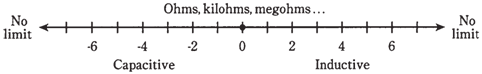## Capacitive reactance Assignment Help

Assignment Help: >> Electrical Engineering - Capacitive reactance

Capacitive reactance

INDUCTIVE REACTANCE  IS LIKE RESISTANCE, IN THE SENSE THAT IT is a 1-dimensional,  or scalar, quantity which can vary from zero upwards without limit. Inductive reactance, like resistance, is represented by a ray, and can be measured in ohms.

Inductive reactance has the counterpart of in form of capacitive reactance. This too can be denoted as a ray, starting at same zero point as the inductive reactance, but running off in opposite direction, having negative ohmic values. When the ray for the capacitive reactance is combined with ray for inductive reactance, a number line is the result, with the ohmic values which range from the huge negative numbers, through zero, to the huge positive numbers.Figure-   Inductive and capacitive reactance can be represented on the complete ohmic number line.

 Capacitors and alternating current Capacitors and direct current Current leads voltage Determine the lead Determine the lead by trigonometric method Points in the RC plane Reactance and frequency Vectors in the RC plane